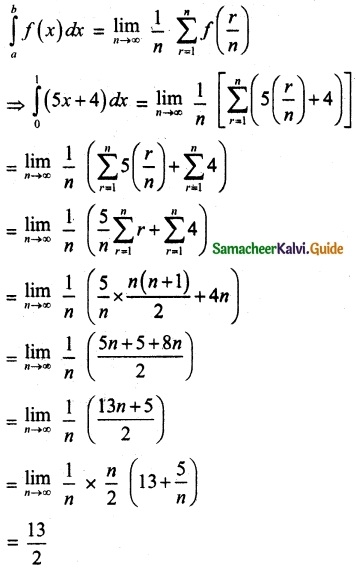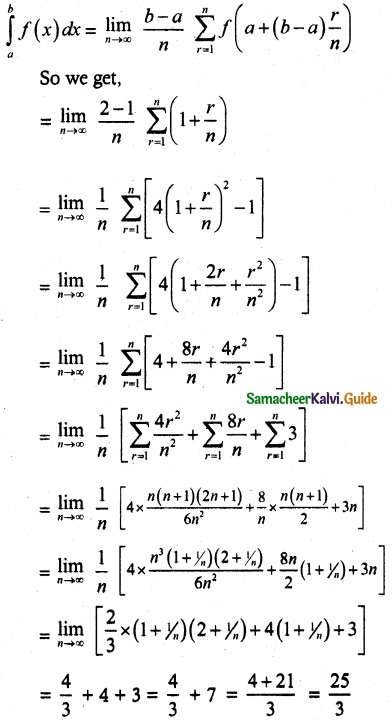Tamilnadu State Board New Syllabus Samacheer Kalvi 12th Maths Guide Pdf Chapter 9 Applications of Integration Ex 9.2 Textbook Questions and Answers, Notes.

## Tamilnadu Samacheer Kalvi 12th Maths Solutions Chapter 9 Applications of Integration Ex 9.2

Question 1.
Evaluate the following integrals as the limits of sums:
(i) $$\int_{0}^{1}$$ (5x + 4)dx
Solution:
(i) Here f(x) = 5x + 4, a = 0, b = 1
Hence we get(ii) $$\int_{1}^{2}$$ (4x² – 1)dx
Solution:
Here f(x) = 4x² – 1, a = 1, b = 2
We use the formula